##### Backtesting with thinkscript

I cobbled together a bunch of examples I found through Google into the samples provided below. thinkorswim is not that great for this, but it does allow you to visualize trades and get some idea of how well a strategy works. However, there is no way to fully auto trade a strategy. It’s possible to trigger an order based on a strategy being “true” but I would not recommend doing so. One thing to note is the sound alerts do not work and I haven’t found a solution.

Limitations

1. Strategy can only be backtested on the chart duration it is applied to. This means a full backtest of years worth if intraday data is not possible unless you use the “OnDemand” feature.
2. Strategy results window only shows basic trade P/L and a total P/L. No information about winning percentage or useful averages.
3. Targets/stops end up trailing if the value changes (ATR in this case) and I haven’t found a way to make them static. I found examples to do that but they never worked properly.

Warning about stops. If you start seeing drastic improvements to your algos losses, make sure thinkorswim did not remove the `price` field from the stop loss order. This seems to occur when you save the strategy and it contains an error.

## Creating a Strategy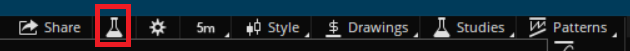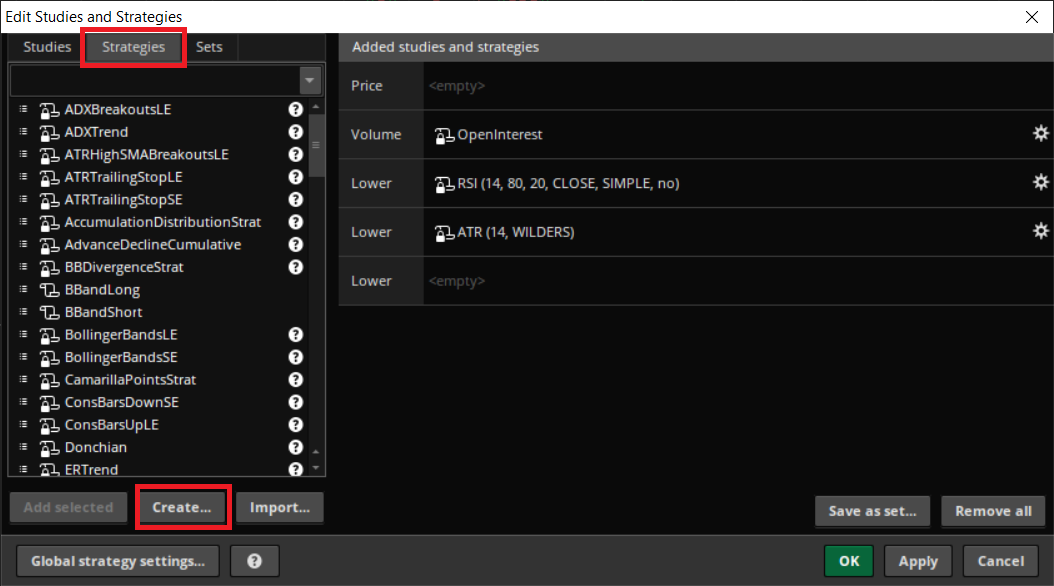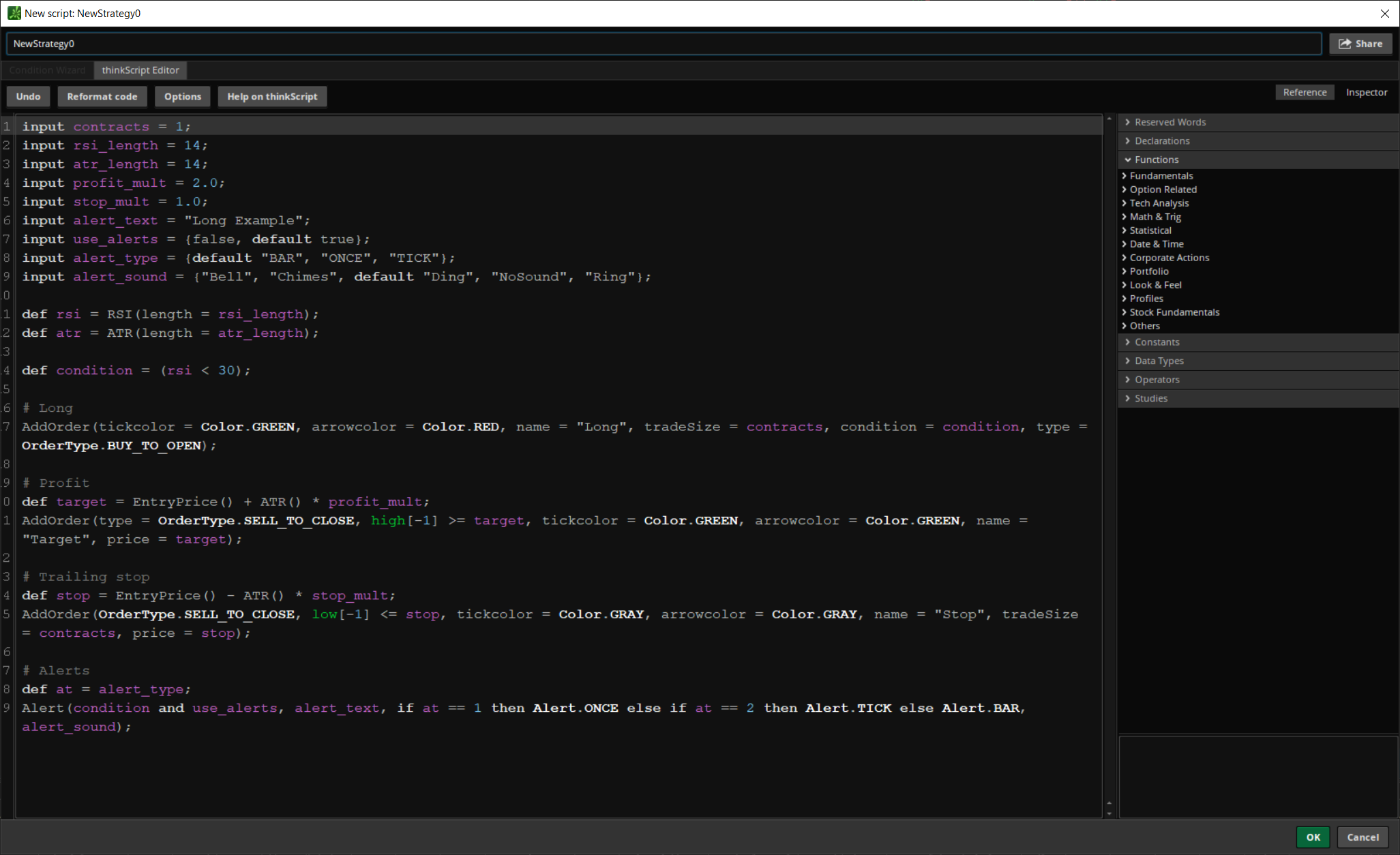The strategy will now appear in the “Price” window.You should now see some trades on the chart. If not, it means your strategy has no trades or there’s something wrong with your stop loss/profit target prices causing it to only show up one time.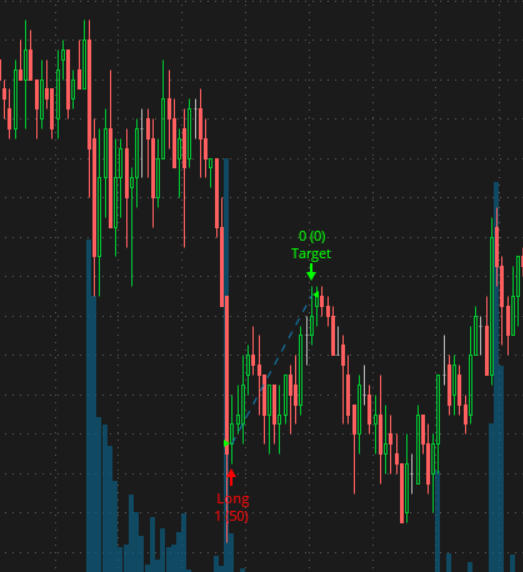## Backtesting

Now that you have a test strategy that shows up on the chart, you can backtest it to see the P/L. The first thing you will want to do is change the timeframe to as far back as possible. On the 5m, thinkorswim only allows you to go back 180 days. You can experiment with different timeframes and using the “OnDemand” feature to go back to certain years.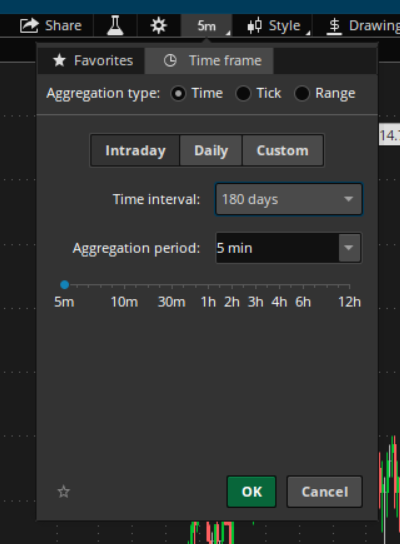Right click on any entry, target, or stop and go to “Show report”.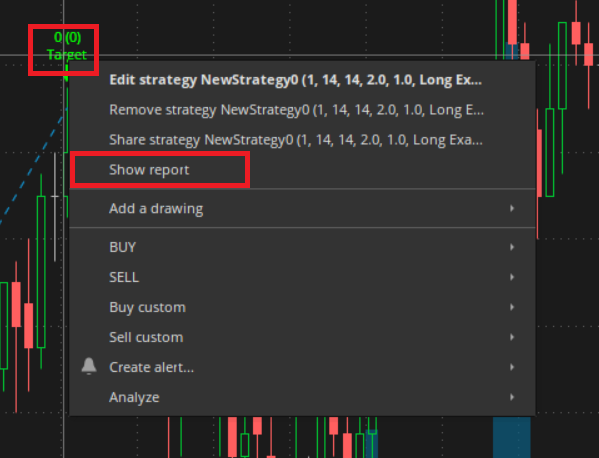And here are the results of the “Long Example” below starting on 4/13/19 going back 180 days on the 5m chart.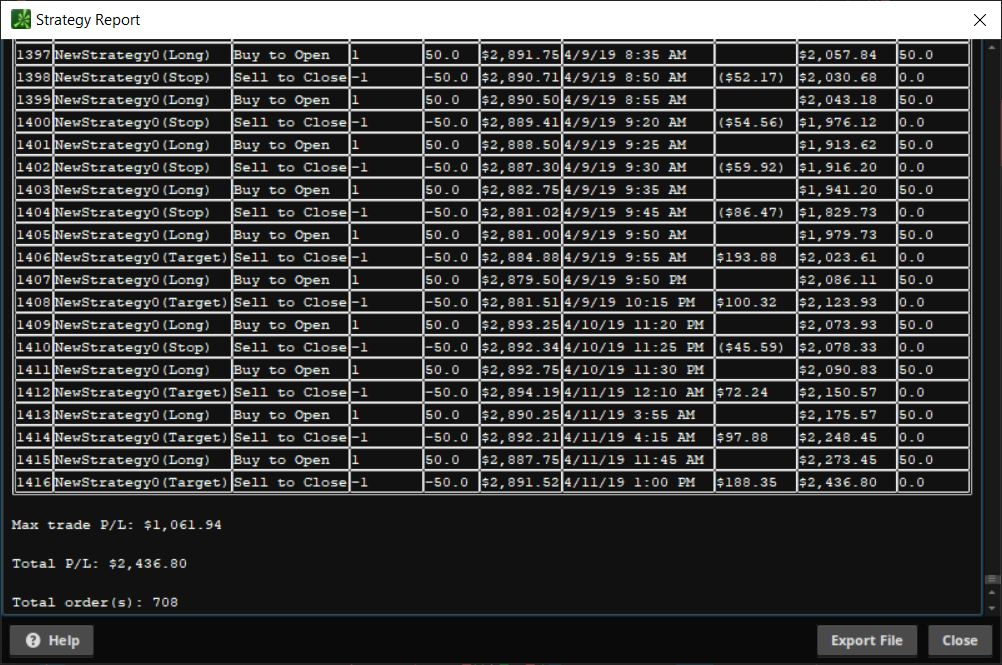The strategy technically made money, however, it’s wildly inconsistent. Just because it made money in the end does not make it worth trading.

## Long Example

``````input contracts = 1;
input rsi_length = 14;
input atr_length = 14;
input profit_mult = 2.0;
input stop_mult = 1.0;
input use_alerts = {false, default true};
input alert_type = {default "BAR", "ONCE", "TICK"};
input alert_sound = {"Bell", "Chimes", default "Ding", "NoSound", "Ring"};

def rsi = RSI(length = rsi_length);
def atr = ATR(length = atr_length);

def condition = (rsi < 30);

# Long
AddOrder(tickcolor = Color.GREEN, arrowcolor = Color.RED, name = "Long", tradeSize = contracts, condition = condition, type = OrderType.BUY_TO_OPEN);

# Profit
def target = EntryPrice() + ATR() * profit_mult;
AddOrder(type = OrderType.SELL_TO_CLOSE, high[-1] >= target, tickcolor = Color.GREEN, arrowcolor = Color.GREEN, name = "Target", price = target);

# Trailing stop
def stop = EntryPrice() - ATR() * stop_mult;
AddOrder(OrderType.SELL_TO_CLOSE, low[-1] <= stop, tickcolor = Color.GRAY, arrowcolor = Color.GRAY, name = "Stop", tradeSize = contracts, price = stop);

``````

## Short Example

``````input contracts = 1;
input rsi_length = 14;
input atr_length = 14;
input profit_mult = 2.0;
input stop_mult = 1.0;
input use_alerts = {false, default true};
input alert_type = {default "BAR", "ONCE", "TICK"};
input alert_sound = {"Bell", "Chimes", default "Ding", "NoSound", "Ring"};

def rsi = RSI(length=rsi_length);
def atr = ATR(length=atr_length);

def condition = (rsi > 70);

# Short
AddOrder(tickcolor = Color.GREEN, arrowcolor = Color.RED, name = "Short", tradeSize = contracts, condition = condition, type = OrderType.SELL_TO_OPEN);

# Profit
def target = EntryPrice() - ATR() * profit_mult;
AddOrder(type = OrderType.BUY_TO_CLOSE, low[-1] <= target, tickcolor = Color.GREEN, arrowcolor = Color.GREEN, name = "Target", price = target);

# Trailing stop
def stop = EntryPrice() + ATR() * stop_mult;
AddOrder(OrderType.BUY_TO_CLOSE, high[-1] >= stop, tickcolor = Color.GRAY, arrowcolor = Color.GRAY, name = "Stop", tradeSize = contracts, price = stop);

``````

``````input Size = 100;
input OpenTime = 0930;
input CloseTime = 1600;

def condition = SecondsFromTime(CloseTime) == 0;

AddOrder(type = OrderType.BUY_AUTO, tickcolor = Color.GREEN, arrowcolor = Color.RED, name = "Long", tradeSize = Size, condition = condition, price = dailyClose);

# Sell
AddOrder(type = OrderType.SELL_TO_CLOSE, SecondsFromTime(OpenTime) == 0, tickcolor = Color.GREEN, arrowcolor = Color.GREEN, name = "Target", price = dailyOpen);
``````

``````input Size = 100;
input OpenTime = 0930;
input CloseTime = 1600;

def condition = SecondsFromTime(OpenTime) == 0;

AddOrder(type = OrderType.BUY_AUTO, tickcolor = Color.GREEN, arrowcolor = Color.RED, name = "Long", tradeSize = Size, condition = condition, price = dailyOpen);

# Sell
AddOrder(type = OrderType.SELL_TO_CLOSE, SecondsFromTime(CloseTime) == 0, tickcolor = Color.GREEN, arrowcolor = Color.GREEN, name = "Target", price = dailyClose);
``````

Some additions you could make to these is seeing if it closed red or closed green on the day.

``````def dailyOpen = open(period = AggregationPeriod.DAY);
close > dailyOpen
``````

or buy only on specific days of the week:

``````def day = GetDayofWeek(GetYYYYMMDD());
(day == 1 or day == 5)
``````

## Stops

Checking for a double top:

``````high == high
``````

Checking for a double bottom:

``````low == low
``````

Ticks for a stop loss:

Add/subtract the tick size from the entry price depending on a long/short trade.

``````def stop = EntryPrice() + (TickSize() * 4);
``````

## Filtering Open/Close Times

``````input OpenTime = 0930;
input CloseTime = 1600;

# Between open/close time
def timeframe = SecondsTillTime(OpenTime) <= 0 and SecondsTillTime(CloseTime) >= 0;

# Outside open/close time
def timeframe = (SecondsTillTime(OpenTime) > 0 or SecondsTillTime(CloseTime) < 0);
``````

Disclaimer

I am not a registered financial adviser/broker/anything. Use this information for entertainment/informational purposes only. Any tickers mentioned are not recommendations to buy/sell/or sell short. They are used as examples only.

Please remember that past performance may not be indicative of future results.